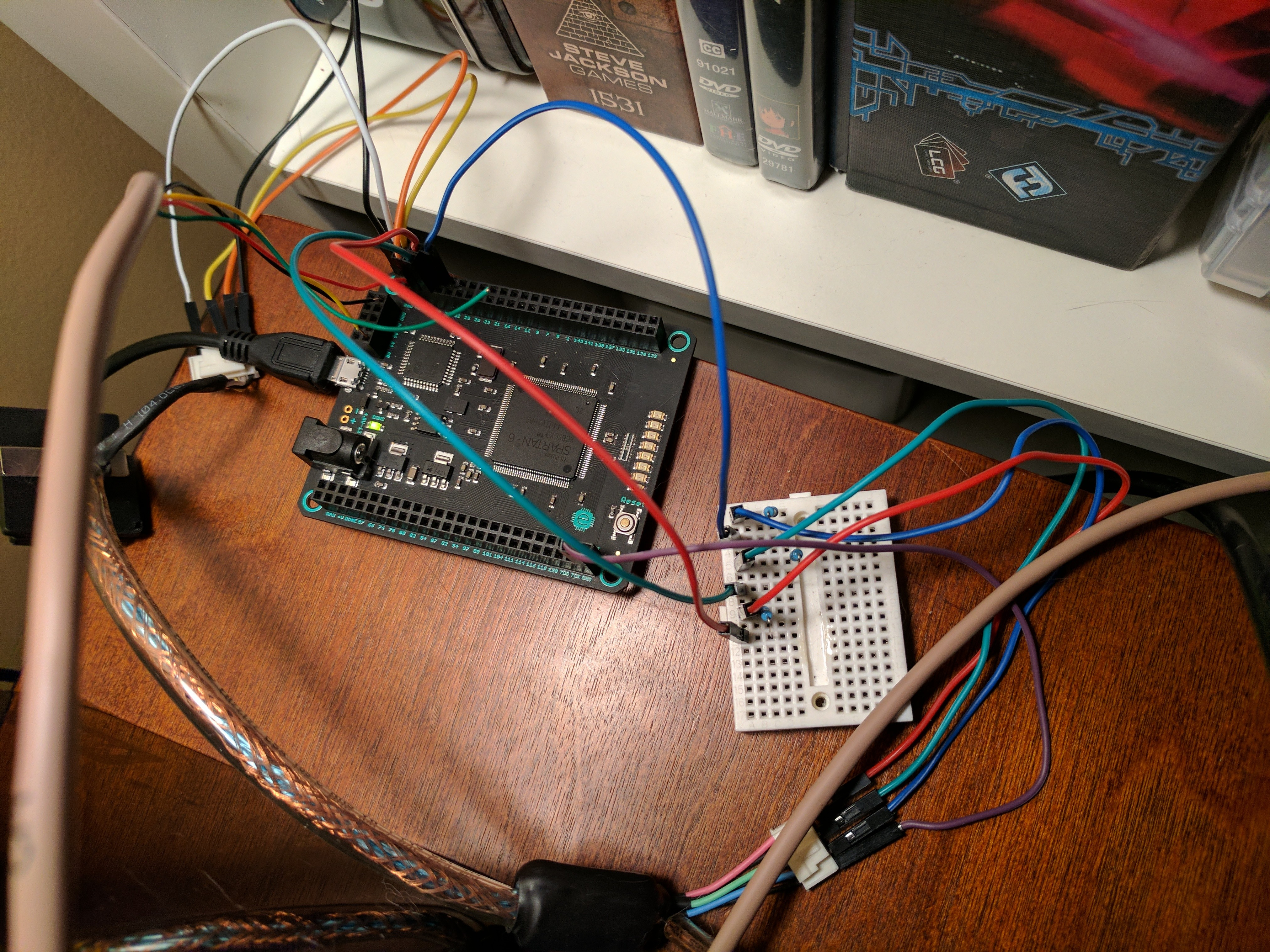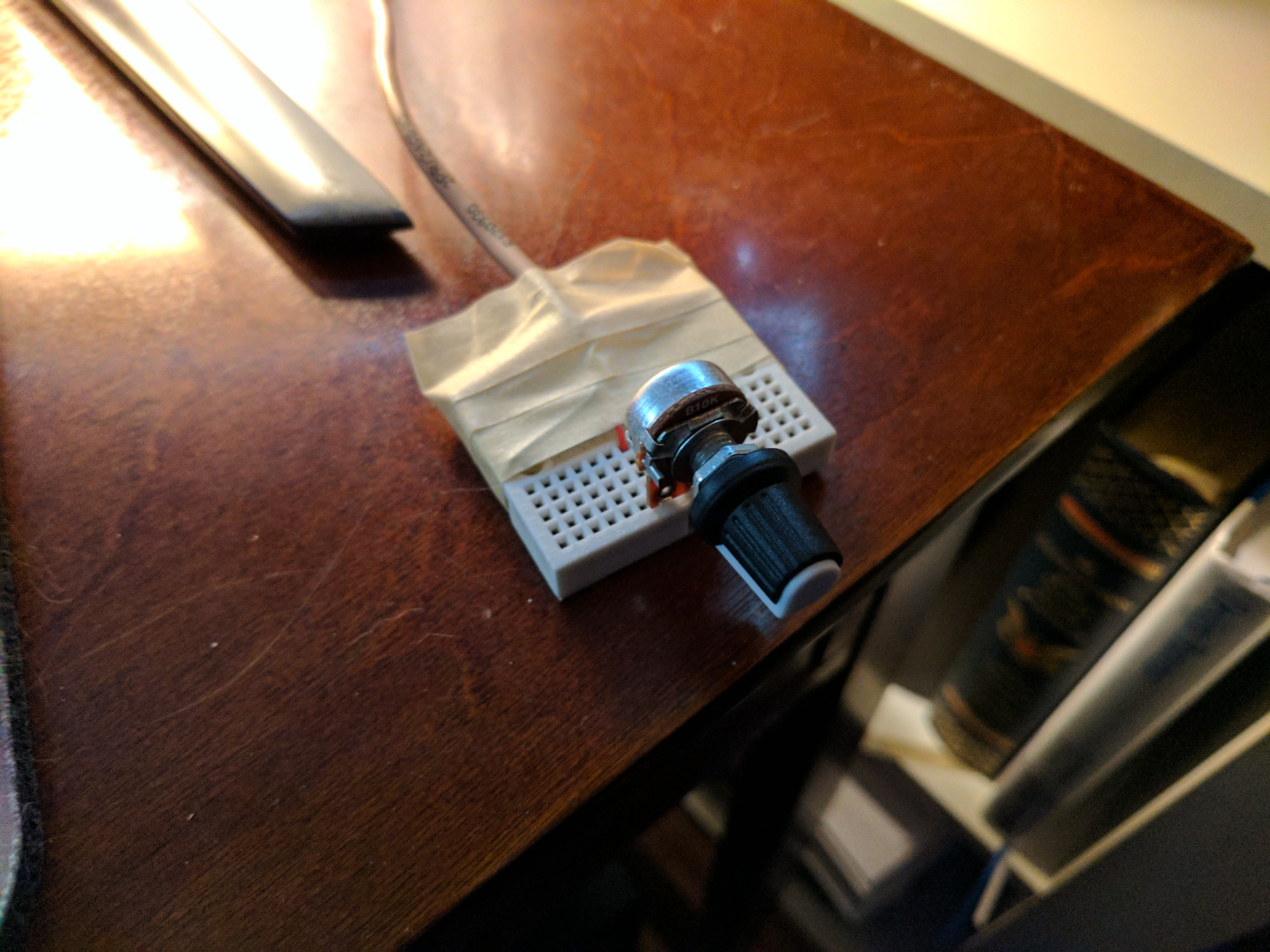# Creating PONG on the Mojo FPGA

I recently found an old VGA monitor on the side of the road and it inspired me to see if I could get my Mojo FPGA board to output a VGA signal. I took the monitor apart and wired its VGA cable directly to my Mojo.

I read up on the VGA specification and learned that getting it to work was as easy as adding resistors to the Red, Green, and Blue outputs.I decided to try my hand at implementing the PONG game that is outlined on fpga4fun.com.

There were some differences between that FPGA that they were using and my Mojo board, so I had to go through some trial-and-error.

For instance, the Verilog code that they have on the site depends on a 25MHz clock and my Mojo uses a 50MHz clock. I tried various ways of dividing the clock without success.

I finally found a great example using a 50MHz clock, but it wasn't quite right because it used 8-bits to encode the RGB values, 3 bits for red and green, and 2 bits for blue. I couldn't get this to work, so I found the code that it was based on and found 1 bit per color implementation. I was finally able to get a signal.

The rest of the time was spent implementing the Pong code from the original article. However, the article used a mouse input. I wanted to use a regular potentiometer (dial) to make it more like the "good old days". So, I wired up a pot to the Mojo and altered the Verilog to use it.

Honestly, my code turned out to be spaghetti code because I haven't quite learned how to modularize Verilog to my satisfaction.

I was able to get the paddle working and responding to movement of the potentiometer, but for some reason, the code for the ball just didn't work in my implementation. I'm guessing it has to do with the timing. I still haven't been able to get the ball working, but I was pretty happy that the paddle works.The Mojo wired to the VGA cable and the paddle "controller"The paddle "controller" - a simple 10k potentiometer

Here is the Verilog definition for the Pong module:

```````timescale 1ns / 1ps
module pong(clk, red, green, blue, hsync, vsync, pot_sample);

// Input clk, 50 MHz Oscillator
input clk;
input [9:0]pot_sample;

// VGA outputs
output red;
output green;
output blue;
output hsync;
output vsync;

reg [9:0] hcount;     // VGA horizontal counter
reg [9:0] vcount;     // VGA vertical counter
reg [2:0] data;		  // RGB data

wire hcount_ov;
wire vcount_ov;
wire inDisplayArea;
wire hsync;
wire vsync;
reg  vga_clk;

// VGA mode parameters
parameter hsync_end   = 10'd95,
hdat_begin  = 10'd143,
hdat_end  = 10'd783,
hpixel_end  = 10'd799,
vsync_end  = 10'd1,
vdat_begin  = 10'd34,
vdat_end  = 10'd514,
vline_end  = 10'd524;

always @(posedge clk)
begin
vga_clk = ~vga_clk;
end

always @(posedge vga_clk)
begin
if (hcount_ov)
hcount <= 10'd0;
else
hcount <= hcount + 10'd1;
end

assign hcount_ov = (hcount == hpixel_end);

always @(posedge vga_clk)
begin
if (hcount_ov)
begin
if (vcount_ov)
vcount <= 10'd0;
else
vcount <= vcount + 10'd1;
end
end
assign  vcount_ov = (vcount == vline_end);

////////////////////////////////////////////////////////////////
// Pong
////////////////////////////////////////////////////////////////

always @(posedge vga_clk) begin

if(0)
begin
if(0)
begin
if(~&PaddlePosition)        // make sure the value doesn't overflow
end
else
begin
if(|PaddlePosition)        // make sure the value doesn't underflow
end
end
end

wire paddle = (hcount >= PaddlePosition + 8) && (hcount <= PaddlePosition+120) && ((vcount <= vdat_end - 15) && (vcount > vdat_end - 25));

// Draw a border around the screen
wire border = ((hcount >=  hdat_begin) && (hcount <  hdat_begin + 10)) || ((hcount >  hdat_end - 10) && (hcount <=  hdat_end))
|| ((vcount >= vdat_begin) && (vcount < vdat_begin + 10)) || ((vcount > vdat_end - 10) && (vcount <= vdat_end));

//////////////////////////////////////////////////////////////////
reg [9:0] ballX;
reg [9:0] ballY;
reg ball_inX, ball_inY;

always @(posedge vga_clk)
if(ball_inX==0) ball_inX <= ((hcount>ballX) && (hcount<=ballX+16)) & ball_inY; else ball_inX <= 0;//!(hcount==ballX+16);

always @(posedge vga_clk)
if(ball_inY==0) ball_inY <= ((vcount>ballY) && (vcount<=ballY+16)); else ball_inY <= 0;//!(vcount==ballY+16);

wire ball = ball_inX & ball_inY;

/////////////////////////////////////////////////////////////////
wire BouncingObject = border | paddle; // active if the border or paddle is redrawing itself

reg ResetCollision;
always @(posedge vga_clk) ResetCollision <= (vcount==0) & (hcount==0);  // active only once for every video frame

reg CollisionX1, CollisionX2, CollisionY1, CollisionY2;
always @(posedge vga_clk) if(ResetCollision) CollisionX1<=0; else if(BouncingObject & (hcount==ballX   ) & (vcount==ballY+ 8)) CollisionX1<=1;
always @(posedge vga_clk) if(ResetCollision) CollisionX2<=0; else if(BouncingObject & (hcount==ballX+16) & (vcount==ballY+ 8)) CollisionX2<=1;
always @(posedge vga_clk) if(ResetCollision) CollisionY1<=0; else if(BouncingObject & (hcount==ballX+ 8) & (vcount==ballY   )) CollisionY1<=1;
always @(posedge vga_clk) if(ResetCollision) CollisionY2<=0; else if(BouncingObject & (hcount==ballX+ 8) & (vcount==ballY+16)) CollisionY2<=1;

/////////////////////////////////////////////////////////////////
wire UpdateBallPosition = ResetCollision;  // update the ball position at the same time that we reset the collision detectors

reg ball_dirX, ball_dirY;
always @(posedge vga_clk)
if(UpdateBallPosition)
begin
if(~(CollisionX1 & CollisionX2))        // if collision on both X-sides, don't move in the X direction
begin
ballX <= ballX + (ball_dirX ? -1 : 1);
if(CollisionX2) ball_dirX <= 1; else if(CollisionX1) ball_dirX <= 0;
end

if(~(CollisionY1 & CollisionY2))        // if collision on both Y-sides, don't move in the Y direction
begin
ballY <= ballY + (ball_dirY ? -1 : 1);
if(CollisionY2) ball_dirY <= 1; else if(CollisionY1) ball_dirY <= 0;
end
end

/////////////////////////////////////////////////////////////////

// display area calculation
&& ((vcount >= vdat_begin) && (vcount < vdat_end));

assign hsync = (hcount > hsync_end);
assign vsync = (vcount > vsync_end);

reg vga_R, vga_G, vga_B;

always @(posedge vga_clk)
begin
vga_R <= BouncingObject | ball;
vga_G <= BouncingObject | ball;
vga_B <= BouncingObject | ball;
end

assign red = (inDisplayArea) ?  vga_R : 0;
assign green = (inDisplayArea) ?  vga_G : 0;
assign blue = (inDisplayArea) ?  vga_B : 0;

// generate "image"
always @(posedge vga_clk)
begin
data <= (vcount[2:0] ^ hcount[2:0]);
end

endmodule``````

Line #6 is the direct input of the potentiometer from the Mojo's on-board Analog to Digital Converter (ADC). Here is the top file showing how it's all wired together:

``````module mojo_top(
// 50MHz clock input
input clk,
// Input from reset button (active low)
input rst_n,
// cclk input from AVR, high when AVR is ready
input cclk,
// Outputs to the 8 onboard LEDs
output[7:0]led,
// AVR SPI connections
output spi_miso,
input spi_ss,
input spi_mosi,
input spi_sck,
output [3:0] spi_channel,
// Serial connections
input avr_tx, // AVR Tx => FPGA Rx
output avr_rx, // AVR Rx => FPGA Tx
input avr_rx_busy, // AVR Rx buffer full
output  red,
output  green,
output  blue,
output hsync, vsync
);

wire rst = ~rst_n; // make reset active high

// these signals should be high-z when not used
assign spi_miso = 1'bz;
assign avr_rx = 1'bz;
assign spi_channel = 4'bzzzz;

assign led = 8'b0;

wire [3:0] channel;
wire new_sample;
wire [9:0] sample;
wire [3:0] sample_channel;
wire [9:0] pot_sample;

avr_interface avr_interface (
.clk(clk),
.rst(rst),
.cclk(cclk),
.spi_miso(spi_miso),
.spi_mosi(spi_mosi),
.spi_sck(spi_sck),
.spi_ss(spi_ss),
.spi_channel(spi_channel),
.tx(avr_rx),
.rx(avr_tx),
.channel(channel),
.new_sample(new_sample),
.sample(sample),
.sample_channel(sample_channel),
.tx_data(8'h00),
.new_tx_data(1'b0),
.tx_busy(),
.tx_block(avr_rx_busy),
.rx_data(),
.new_rx_data()
);

input_capture input_capture (
.clk(clk),
.rst(rst),
.channel(channel),
.new_sample(new_sample),
.sample(sample),
.sample_channel(sample_channel),
.sample_out(pot_sample)
);

pong pongBoard(
.clk(clk),
.red(red),
.green(green),
.blue(blue),
.hsync(hsync),
.vsync(vsync),
.pot_sample(pot_sample)
);

endmodule``````

And, finally, here are the custom user constraints that I used to wire the output pins up to the VGA connector:

``````NET "hsync" LOC = P50 | IOSTANDARD = LVTTL;
NET "vsync" LOC = P51 | IOSTANDARD = LVTTL;
NET "red" LOC = P41 | IOSTANDARD = LVTTL;
NET "green" LOC = P40 | IOSTANDARD = LVTTL;
NET "blue" LOC = P35 | IOSTANDARD = LVTTL;``````

I'd really like to get the ball working with this. My scoring will be different than traditional Pong as I am not yet ready to tackle the "AI" of the computer player. I'd like the player to score 1 point each time the ball hits the paddle and lose a point each time the ball hits the bottom wall. If the ball hits the bottom wall while the score is 0, then the game is over. I'll have to add in a reset button, but that shouldn't be much trouble.

I just found another implementation of Pong in VHDL that I may try later. It divides the 50MHz clock into a 25MHz clock using a mod 2 counter. I discuss my implementation of that code in another post.

I haven't written any VHDL, yet, so I'm still trying to wrap my head around it. It was hard enough switching from Mojo's Lucid language to Verilog as Lucid made things a bit easier. However, I felt that since there were a lot more people out there using Verilog that I would be better off learning that. Now I'm learning that there are even more people using VHDL.

Lucid, Verilog, and VHDL are a far cry from C#, C, and JavaScript; three languages I'm very familiar with. This has been a very beneficial lesson in parallel "programming".